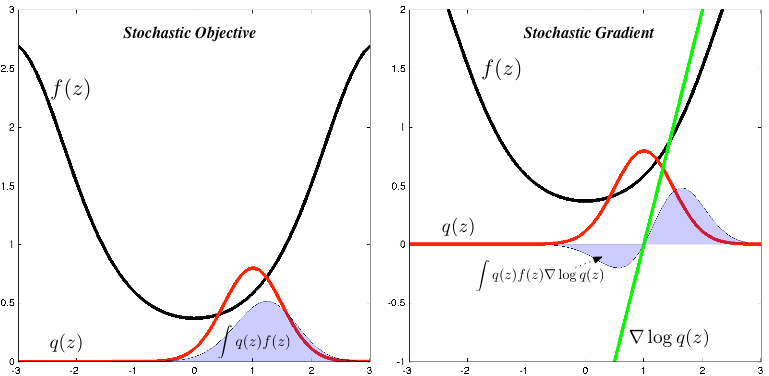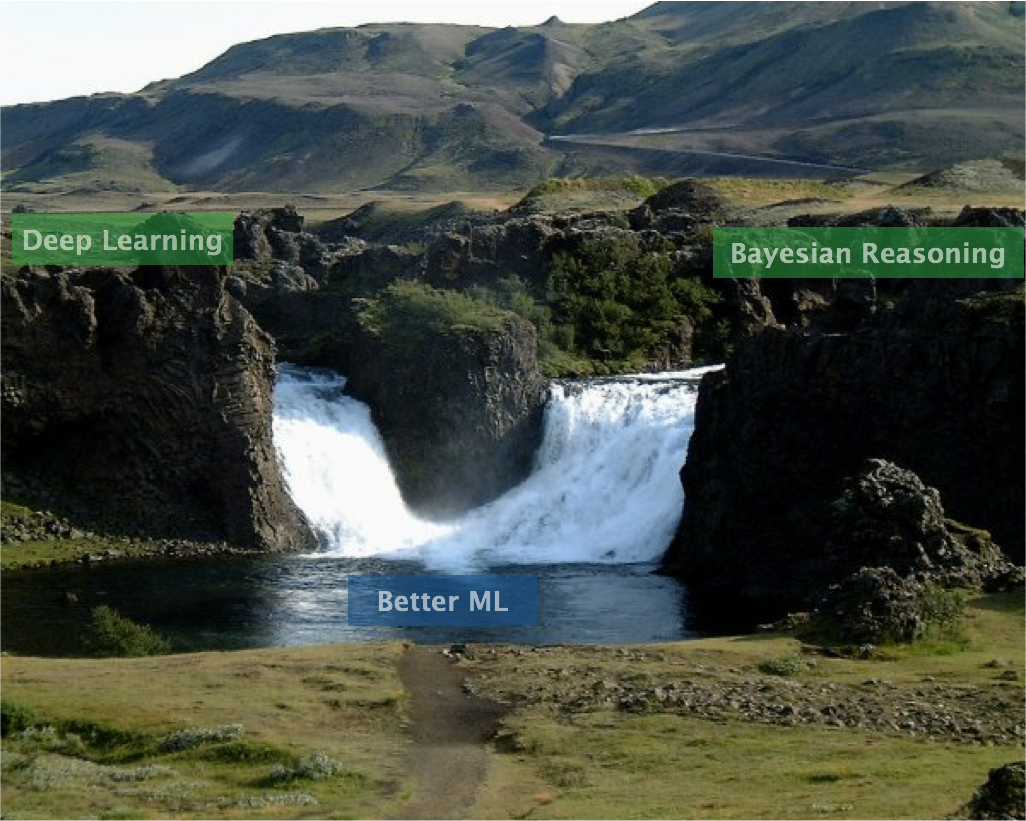# Machine Learning Trick of the Day (5): Log Derivative Trick

Machine learning involves manipulating probabilities. These probabilities are most often represented as normalised-probabilities or as log-probabilities. An ability to shrewdly alternate between these two representations is a vital step towards strengthening the probabilistic dexterity we need to solve modern machine learning problems. Today's trick, the log derivative trick, helps … Continue reading Machine Learning Trick of the Day (5): Log Derivative Trick# Talk: Memory-based Bayesian Reasoning and Deep Learning

A talk that explores the convergence of deep learning and Bayesian inference.  We'll take a statistical tour of deep learning, think about approximate Bayesian inference, and explore the idea of doing inference-with-memory and the different ways that this manifests itself in … Continue reading Talk: Memory-based Bayesian Reasoning and Deep Learning